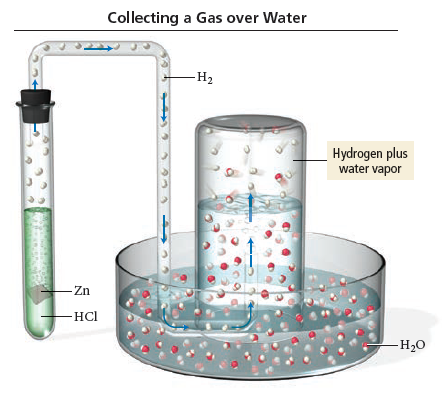# Problem: A common way to make hydrogen gas in the laboratory is to place a metal such as zinc in hydrochloric acid (see the figure). The hydrochloric acid reacts with the metal to produce hydrogen gas, which is then collected over water. Suppose a student carries out this reaction and collects a total of 150.2 mL of gas at a pressure of 746 mmHg and a temperature of 25oC. What mass of hydrogen gas (in mg) does the student collect? (The vapor pressure of water is 23.78 mmHg at 25oC.)

###### FREE Expert Solution

Dalton’s Law states that the total pressure inside a container is obtained by adding all the partial pressures of each non-reacting gas

${\mathbit{P}}_{{\mathbf{H}}_{\mathbf{2}}}\mathbf{=}$722.22 mm Hg

Ideal gas equation: moles H2

$\frac{\mathbf{PV}}{\mathbf{RT}}\mathbf{=}\frac{\mathbf{n}\overline{)\mathbf{RT}}}{\overline{)\mathbf{RT}}}\phantom{\rule{0ex}{0ex}}\mathbf{n}\mathbf{=}\frac{\mathbf{PV}}{\mathbf{RT}}$

mass = moles x molar mass

86% (479 ratings)###### Problem Details

A common way to make hydrogen gas in the laboratory is to place a metal such as zinc in hydrochloric acid (see the figure). The hydrochloric acid reacts with the metal to produce hydrogen gas, which is then collected over water. Suppose a student carries out this reaction and collects a total of 150.2 mL of gas at a pressure of 746 mmHg and a temperature of 25oC. What mass of hydrogen gas (in mg) does the student collect? (The vapor pressure of water is 23.78 mmHg at 25oC.)Frequently Asked Questions

What scientific concept do you need to know in order to solve this problem?

Our tutors have indicated that to solve this problem you will need to apply the Collecting Gas Over Water concept. If you need more Collecting Gas Over Water practice, you can also practice Collecting Gas Over Water practice problems.

What professor is this problem relevant for?

Based on our data, we think this problem is relevant for Professor Tyson's class at UMASS.